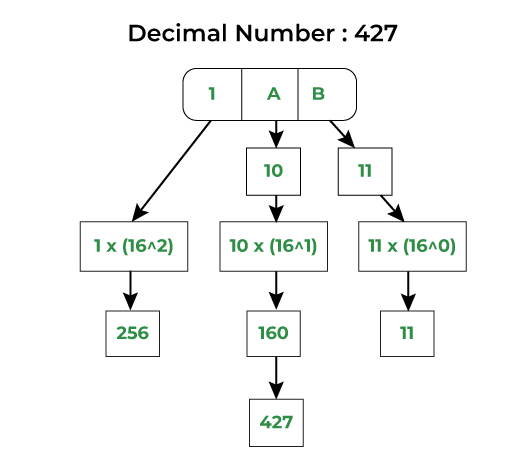# Java Program For Hexadecimal to Decimal Conversion

Given a Hexadecimal number N, convert N into an equivalent decimal number i.e. convert the number with base value 16 to base value 10. The decimal number system uses 10 digits 0-9 and the Hexadecimal number system uses 0-9, A-F to represent any numeric value.

Illustration:

```Input : 1AB
Output: 427
Input : 1A
Output: 26```

## Approach to Convert Hexadecimal to Decimal Conversion

The approach to implementing the conversion is mentioned below:

1. The idea is to extract the digits of a given hexadecimal number starting from the rightmost digit.
2. Keep a variable ‘dec_value’.
3. At the time of extracting digits from the hexadecimal number, multiply the digit with the proper base (Power of 16) and add it to the above variable taken that is ‘dec_value’.
4. In the end, the variable ‘dec_value’ will store the required decimal number.## Programs to Convert Hexadecimal to Decimal Conversion

Below is the implementation of the above approach:

## Java

 `// Java program to convert Hexadecimal  ` `// to Decimal Number ` ` `  `// Importing input output classes ` `import` `java.io.*; ` ` `  `// Main class ` `class` `GFG { ` ` `  `    ``// Method ` `    ``// To convert hexadecimal to decimal ` `    ``static` `int` `hexadecimalToDecimal(String hexVal) ` `    ``{ ` `        ``// Storing the length of the ` `        ``int` `len = hexVal.length(); ` ` `  `        ``// Initializing base value to 1, i.e 16^0 ` `        ``int` `base = ``1``; ` ` `  `        ``// Initially declaring and initializing ` `        ``// decimal value to zero ` `        ``int` `dec_val = ``0``; ` ` `  `        ``// Extracting characters as ` `        ``// digits from last character ` ` `  `        ``for` `(``int` `i = len - ``1``; i >= ``0``; i--) { ` ` `  `            ``// Condition check ` `            ``// Case 1 ` `            ``// If character lies in '0'-'9', converting ` `            ``// it to integral 0-9 by subtracting 48 from ` `            ``// ASCII value ` `            ``if` `(hexVal.charAt(i) >= ``'0'` `                ``&& hexVal.charAt(i) <= ``'9'``) { ` `                ``dec_val += (hexVal.charAt(i) - ``48``) * base; ` ` `  `                ``// Incrementing base by power ` `                ``base = base * ``16``; ` `            ``} ` ` `  `            ``// Case 2 ` `            ``// if case 1 is bypassed ` ` `  `            ``// Now, if character lies in 'A'-'F' , ` `            ``// converting it to integral 10 - 15 by ` `            ``// subtracting 55 from ASCII value ` `            ``else` `if` `(hexVal.charAt(i) >= ``'A'` `                     ``&& hexVal.charAt(i) <= ``'F'``) { ` `                ``dec_val += (hexVal.charAt(i) - ``55``) * base; ` ` `  `                ``// Incrementing base by power ` `                ``base = base * ``16``; ` `            ``} ` `        ``} ` ` `  `        ``// Returning the decimal value ` `        ``return` `dec_val; ` `    ``} ` ` `  `    ``// Method 2 ` `    ``// Main driver method ` `    ``public` `static` `void` `main(String[] args) ` `    ``{ ` `        ``// Custom input hexadecimal number to be ` `        ``// converted into decimal number ` `        ``String hexNum = ``"1A"``; ` ` `  `        ``// Calling the above method to convert and ` `        ``// alongside printing the hexadecimal number ` `        ``System.out.println(hexadecimalToDecimal(hexNum)); ` `    ``} ` `}`

Output

`26`

#### The complexity of the above method

The time complexity is O(n), where n is the length of the input hexadecimal string.
The auxiliary space is O(1), which is constant.

## Algorithm to Convert a Decimal to Binary Number:

There is an algorithm that can be followed to convert a Decimal to Binary Number as mentioned below:

1. Take the input decimal number.
2. If the number is 0, return 0 as the binary equivalent.
3. Initialize an empty string to store the binary digits.
4. Divide the decimal number by 2 and get the quotient and remainder.
5. Convert the remainder to a string and append it to the binary string.
6. Set the decimal number to the quotient obtained in step 4.
7. Repeat steps 4-6 until the decimal number becomes 0.
8. Reverse the binary string obtained in steps 3-7.
9. Return the reversed binary string.

This algorithm uses the division method to convert a decimal number to binary. At each step, the remainder is computed and appended to the binary string. The quotient becomes the new decimal number for the next iteration. Finally, the binary string is reversed to get the correct binary equivalent of the decimal number.

## Java

 `// Java Program for Hexadecimal to ` `// Decimal Conversion ` ` `  `// Driver Class ` `public` `class` `HexToDec { ` `    ``// main function ` `    ``public` `static` `void` `main(String[] args) ` `    ``{ ` `        ``String hex1 = ``"1AB"``; ` `        ``String hex2 = ``"1A"``; ` `        ``int` `dec1 = hexToDec(hex1); ` `        ``int` `dec2 = hexToDec(hex2); ` `        ``System.out.println(hex1 + ``" in decimal is "` `+ dec1); ` `        ``System.out.println(hex2 + ``" in decimal is "` `+ dec2); ` `    ``} ` ` `  `    ``private` `static` `int` `hexToDec(String hex) ` `    ``{ ` `        ``int` `len = hex.length(); ` `        ``int` `dec = ``0``; ` `        ``for` `(``int` `i = ``0``; i < len; i++) { ` `            ``char` `c = hex.charAt(i); ` `            ``int` `digit = Character.digit(c, ``16``); ` `            ``dec = dec * ``16` `+ digit; ` `        ``} ` `        ``return` `dec; ` `    ``} ` `}`

Output

```1AB in decimal is 427
1A in decimal is 26```

#### The complexity of the above method

Time complexity: O(log n), where n is the input decimal number.
Auxiliary Space: O(log n),

Whether you're preparing for your first job interview or aiming to upskill in this ever-evolving tech landscape, GeeksforGeeks Courses are your key to success. We provide top-quality content at affordable prices, all geared towards accelerating your growth in a time-bound manner. Join the millions we've already empowered, and we're here to do the same for you. Don't miss out - check it out now!

Previous
Next